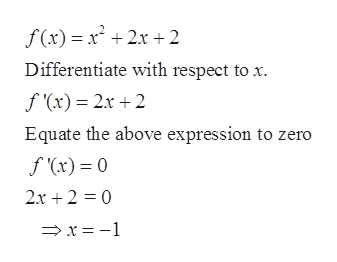Question
3 views
check_circle

Step 1

The given function is f(x) = x2 + 2x + 2

To find the critical v

The given function is f(x) = x2 + 2x + 2

To find the critical values and relative extrema.

alues and relative extrema.

Step 2

First find the derivative of the f(x) and equate it to zero. The solution of equation f’(x) = 0 are the critical values of f(x).help_outlineImage Transcriptionclosef(x) x22x+2 Differentiate with respect to f (x) 2x 2 Equate the above expression to zero f (x)= 0 2x 2 0 x 1 fullscreen
Step 3

Thus x = - 1 is the critical point of...

### Want to see the full answer?

See Solution

#### Want to see this answer and more?

Solutions are written by subject experts who are available 24/7. Questions are typically answered within 1 hour.*

See Solution
*Response times may vary by subject and question.
Tagged in

### Calculus